Typical underground structures include Tunnels, Subway Stations, Underground Parking lots, Culverts, Conduits, etc. These structures should be designed to resist the overburden loads and the seismic load.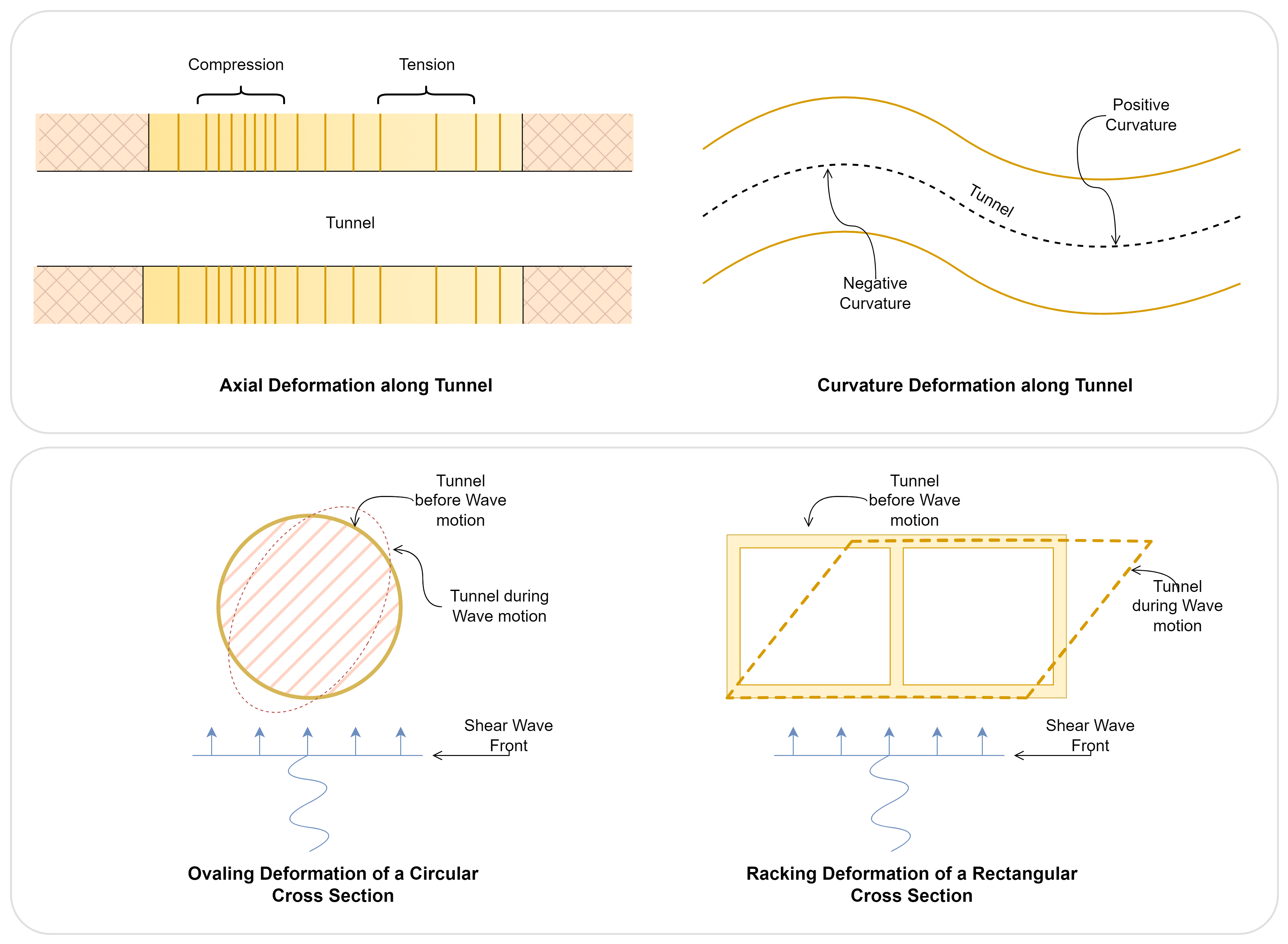Deformations observed in Tunnels and Subways

When considering underground structures, we assume they will not suffer any catastrophic damage from earthquakes as they are completely enclosed by soil. However, in recent news, we learned this is not true due to damaging and failure of underground structures during previous earthquakes.

Underground structures typically have a high construction cost and long-term usage; hence seismic analysis and design are crucial for minimizing risks and damage during an earthquake. The best approach is to ignore the interaction of the structure with soil and apply the free-field deformation to the structure, but Soil-Structure interaction plays a major role. In contrast to above-ground structures where inertia forces are crucial, the deformation of the surrounding soil determines the seismic response of underground structures. Depth of structure, type of soil, relative stiffness of structure and soil, duration of an earthquake, etc., determine the structure's response.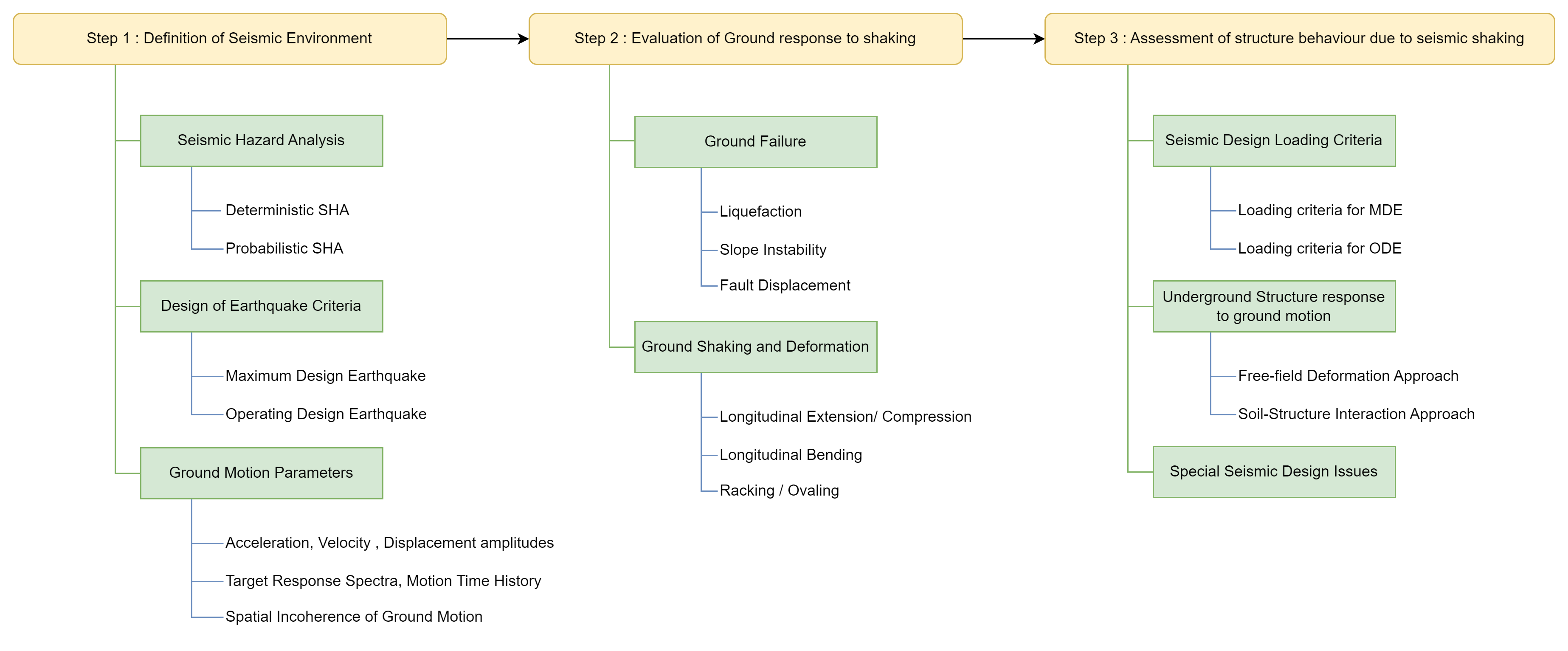Underground Structure Seismic Analysis and Design Procedure

Today we will be going to discuss four methods frequently used for the seismic analysis of underground structures:

• Response Displacement Method
• Equivalent Inertial force method
• Equivalent Horizontal acceleration Method
• Time History Method

Response Displacement Method

Starting with the most used method for the analysis, every engineer must have encountered the Response Spectrum method while studying seismic analysis. The response spectrum shows the maximum response (displacement, velocity, acceleration) versus structural frequency or time period. When the response is displacement, it is termed as Response Displacement curve, and the corresponding method Response Di.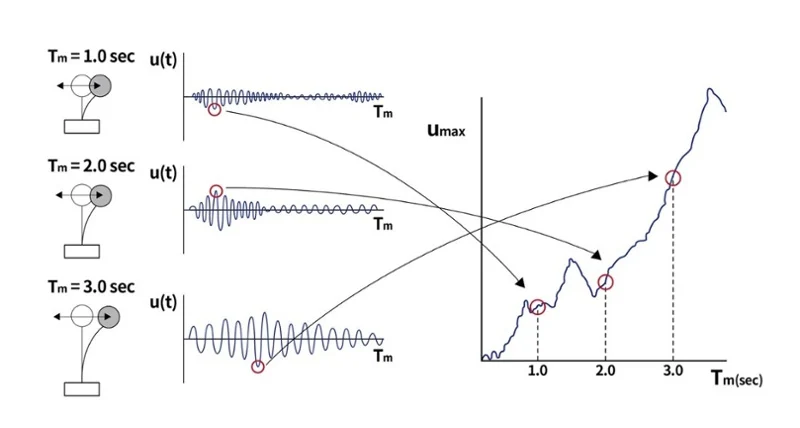Procedure to determine the displacement response spectrum

You might wonder how we get this curve. As shown in the figure above, assume the displacement response of three SDOF systems with constant damping ratio and different natural periods. These systems are then subjected to seismic excitation, and their maximum displacement is noted. The deformation response spectrum can be represented as a function of the natural vibration period, and the maximum displacement of each ground motion system observed.

When designing an underground structure, the focus is on the deformation of the surrounding soil (free-field deformation). This is the reason that instead of going for the usual Response Acceleration method, Response deformation is used.

Due to its clear physical conception and accurate simulation of the mechanical characteristic of structures, this method is employed worldwide. The Response displacement method can truly reflect the underground structure stress characteristics.

Equivalent Inertial Force Method

The Equivalent Inertial Force method is based on the idea that the maximum bending moment values obtained by the two methods—dynamic time-history analysis and equivalent inertial force, are the same and obtained at the same location. To achieve the same outcome as that of dynamic time-history analysis, a correction factor must be applied to calculate the bending moment of the remaining members and the axial force and shear force of all the components.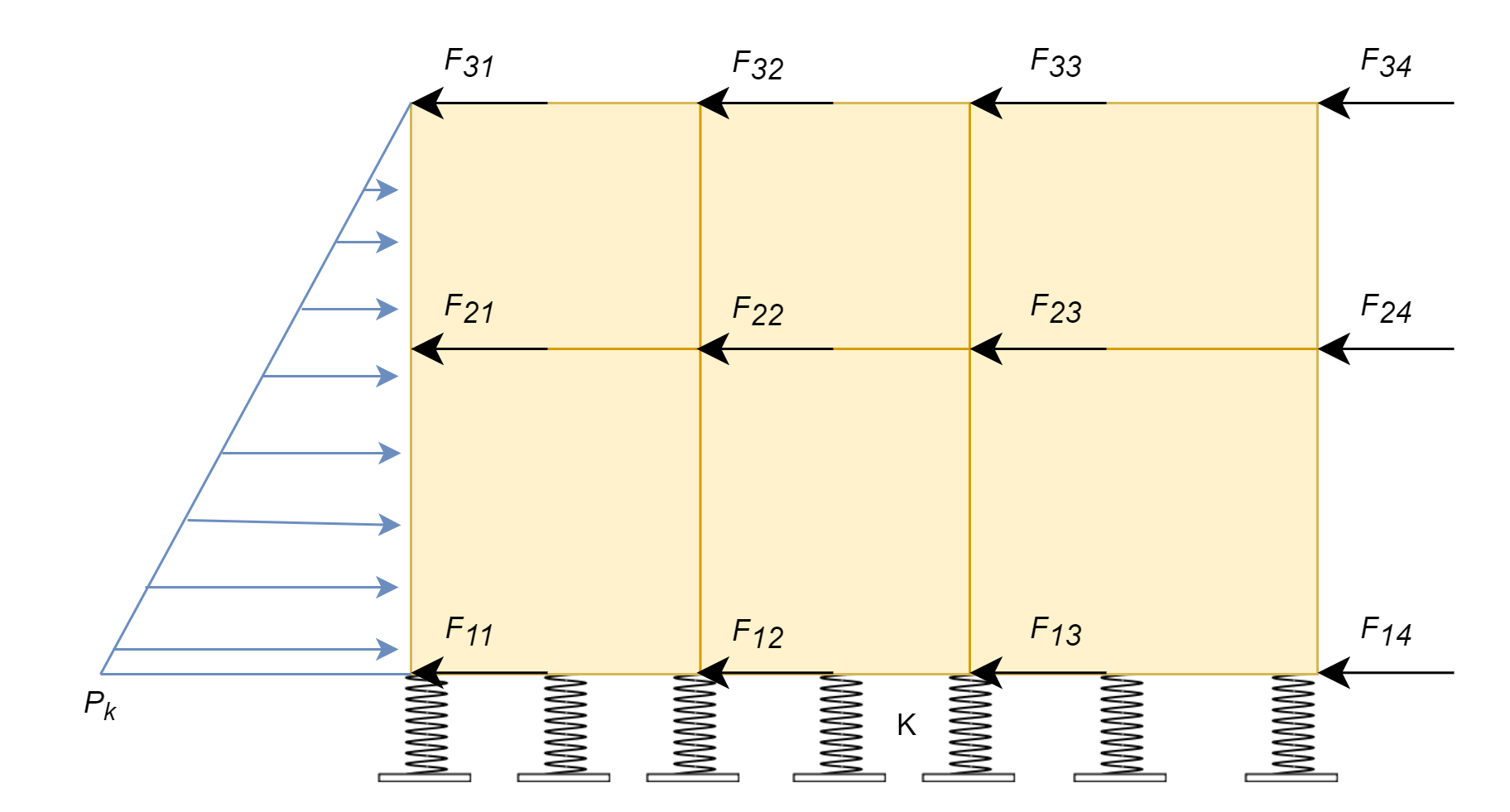Equivalent Inertial Force Method

Let’s see how the equivalent horizontal seismic inertia force is obtained. In the above figure,Where,

F𝑖𝑗 is equivalent horizontal seismic inertia force acting on node 𝑖𝑗

𝑚𝑖𝑗 is half the sum of the mass of the member or simply nodal mass

𝑘 is the equivalent inertial force coefficient.

Calculation of Equivalent Inertial Force Coefficient(k), takes the effect of :

1. Depth of embedment
2. Nature of Foundation Soil
3. Seismic acceleration.

The Shanghai code assumes a triangular horizontal resistance force and employs an internal force correction coefficient to match the results with time-history analysis.

Equivalent Horizontal Acceleration Method

The equivalent horizontal Acceleration method is simplified and replaces the time-history horizontal acceleration with a static horizontal acceleration.

In this method, the basis of the static acceleration distribution pattern is based on the experimental and time-history analysis results. Soft soils have an amplification effect on earthquake acceleration and velocity. The shear and axial forces are determined by introducing a correction factor consistent with the dynamic time history method.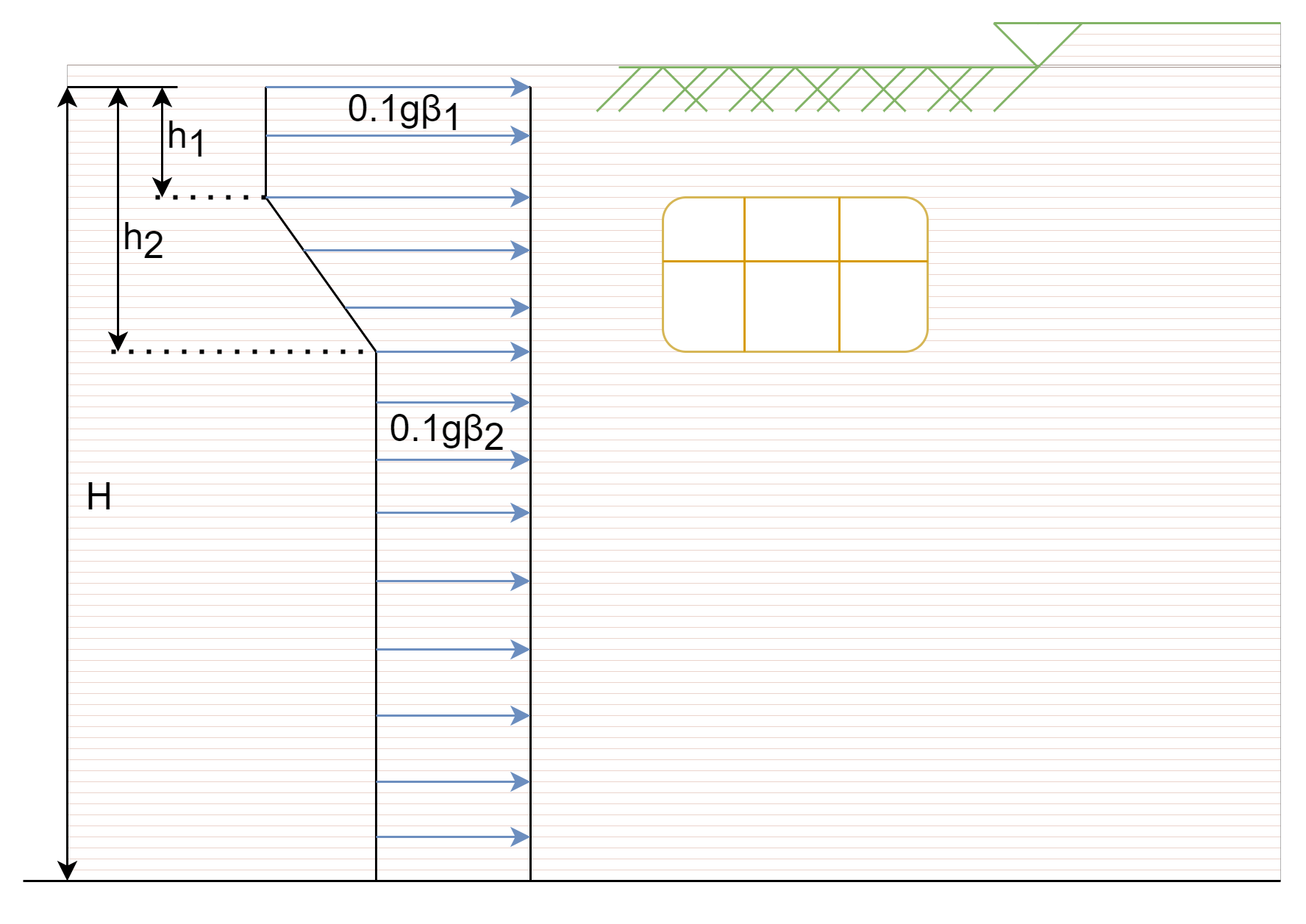Equivalent Horizontal Acceleration Method

In the figure above, H is the total thickness of the soil, h1 depth of the roof slab, and h2 depth of the floor slab.

βand βrepresent the accelerated velocity influence coefficient.

The distribution pattern of static acceleration is based on experimental studies and time-history analysis results. In a nutshell, the Equivalent horizontal acceleration method transforms the dynamic analyses into a static calculation process to improve computational efficiency.

Time History Method

Time history analysis seeks out a solution for the dynamic equilibrium equation when a structure is subjected to dynamic loads. It calculates a series of structural responses (displacements, member forces, etc.) within a given period of time based on the structure's dynamic characteristics under the applied loads.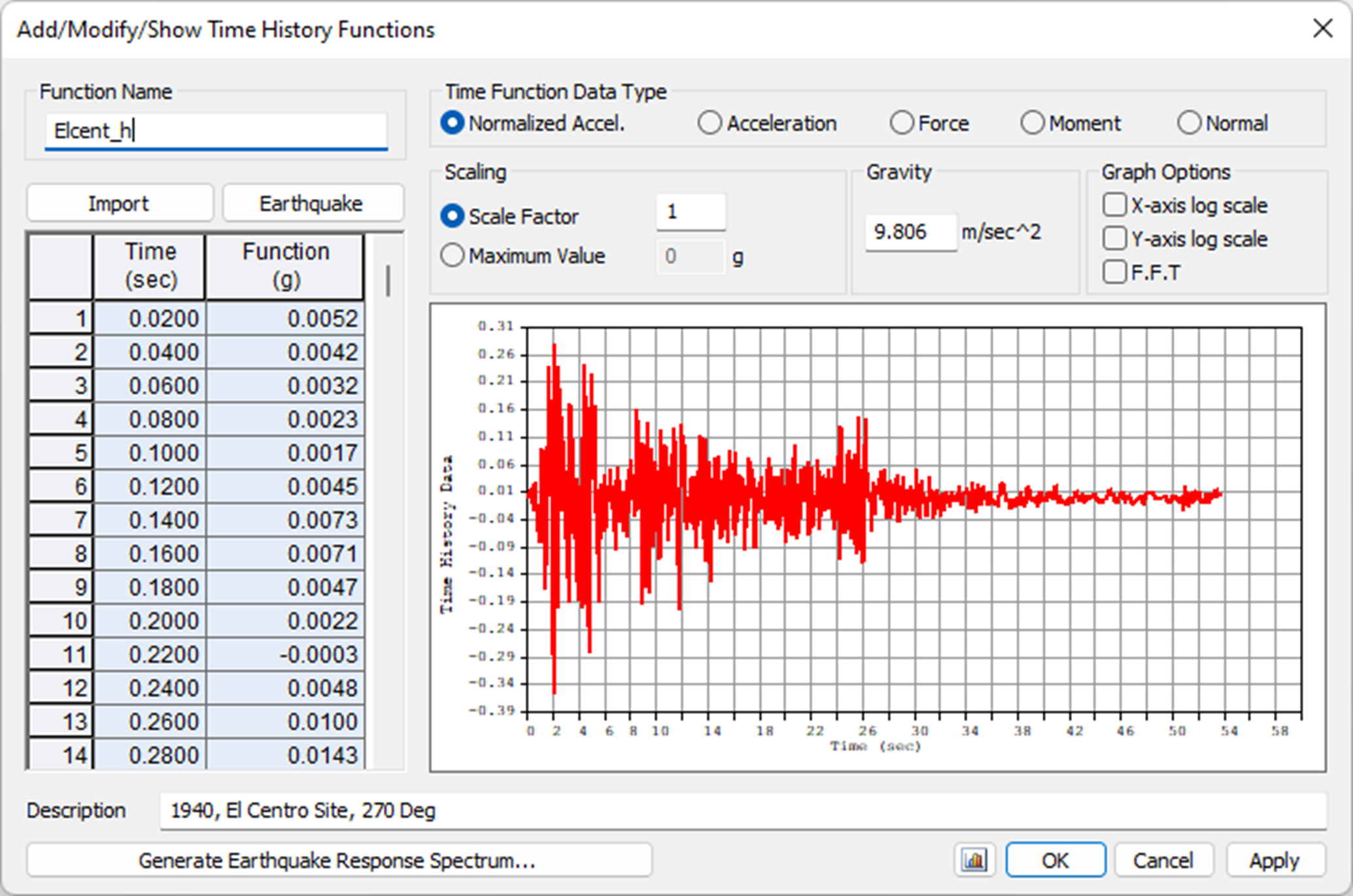Time History Function defined in midas Civil

The dynamic time history analysis approach employed in soft soil layer seismic response analysis of underground structures is extremely consistent with the actual scenario and calculates accurately.

This approach can also accurately reflect the soil and structure interaction during an earthquake. The economy in the design achieved cannot be neglected even though the calculation is complex and time-consuming.

Summary

We are reaching the end of this article. Let’s go back to the beginning to summarize things. There are two approaches to analyzing underground structures:

1. Use time-history analysis on a FEM model. The complete structure, including the soil, is modeled, and the ground motion is applied to the boundaries. This method simulates wave propagation. A lot of calculation is needed, which is too complex and time-consuming.
2. Pseudo-Static Approach. The aim of this approach is to convert dynamic seismic loads into equivalent static loads. This method, though it has a lot of assumptions, makes designing a lot easier and faster.

Of the above-discussed methods, the first three, ie. Response Displacement, Equivalent Inertial Force, and Equivalent Horizontal Acceleration Method come under the pseudo-static approach. These methods assume soil to be linearly elastic and hence apply to the soil under small excitation.

With the recent realization of their importance, countries have started developing and using specialized codes for the seismic analysis of underground structures. Some of the coral provisions are listed below:

Codal Provisions

• Guide Specifications of Design and Construction of Underground Parking Lots, Japan Roadways
• Earthquake Design Criteria for Subways, Thomas R. Kuesel, F. ASCE
• Code for Seismic Design of Subway Structures; China Architecture & Building Press
• (GB/T 51336-2018) Standard for Seismic Design of Underground Structures; China Architecture & Building Press.

Keep Updated With Technical Contents

Keeping You Updated

You might also like...#### The importance of API and its use#### Expert Tip: Concrete shear design based on AASHTO LRFD 2#### Expert Tip: Concrete shear design based on AASHTO LRFD 1